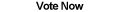Home Articles Components Code Snippets Links# C# Get Image Hash Code

## Bitmap Hash Code

Calculating the hash code of a C# image is useful in many image processing applications. A hash code can be used to compare two images for perfect equality or can be used to make sure an image remains unmodified.

As we will see, calculating the hash code of a bitmap is no different than getting the hash value of any piece of data.

## What is a Hash Code

A hash code is a value generated from a separate starting value (the seed value). A hash code is usually a fixed length for any given size data. So for example, if we are talking about bytes, a seed value that is 1-byte long and a seed value that is 300-bytes long will result in a hash value of the same length.

As you might guess, this means that it is possible for two different seed values to have the same hash value. (There are complicated math proofs for this, but just think about it, it is not too difficult to understand how this happens). When two different seed values result in the same hash value, that is called a collision.

Here is where we introduce the concept of hashing algorithms. A hashing algorithm, as used commonly with data encryption, is a process that takes a seed value and transforms it into a hash value. Good algorithms will use certain math properties to minimize the chance of collisions. Popular algorithms are the MD5 and SHA hashing algorithms. These algorithms are very good at reducing the number of collisions.

Of course, there are a ton of hashing algorithms, some better than others. SHA for example is a family of many different algorithms, not a single algorithm. For those interested in the topic, as of early 2010, there is a public competition to create the new SHA-3 algorithm.

Luckily for C# developers, the .NET Framework comes with implementation of widely-used hashing algorithms.

## Hash Code of an Image

So how do we calculate the hash code of an image? The trick is to remember that an image is stored in a computer in bits, just like any other piece of data. If we can get the bytes that represent the given image, we can calculate the hash value of those bytes. As long as the image-to-byte transformation is consistent, the resulting hash value will always be the same for a given image.

As it so happens, converting a bitmap to bytes is a trivial problem. This article describes two of many ways to turn an image into bytes in C#. Just remember that whatever method you use, you have to make sure it is the same method every time.

Important Note: Every object in .NET has a GetHashCode() function. Some people are tempted to do something like this:

```Bitmap myImage = new Bitmap(10, 10);
int hash = myImage.GetHashCode();```

This will not work. At least not in the purpose of image processing. Why? Because the GetHashCode function will return a hash value for the bitmap object. This is used in C# programming to compare when two references are pointing to the same object (since they will return the same hash value calculated on the object). You might have two separate images that are identical, but because the are each represented by an object, the GetHashCode values will be completely different.

### Page 2Bitmap Hashing Example >>

Back to C# Article List

Other C# Articles